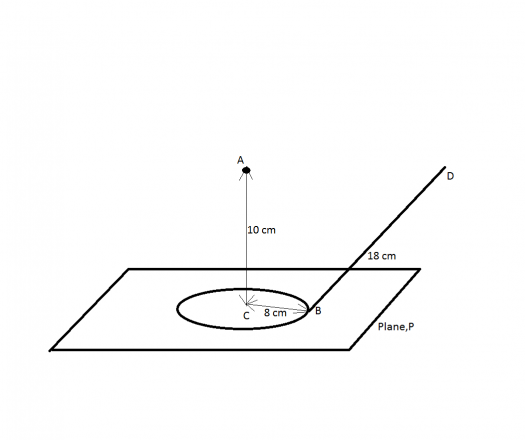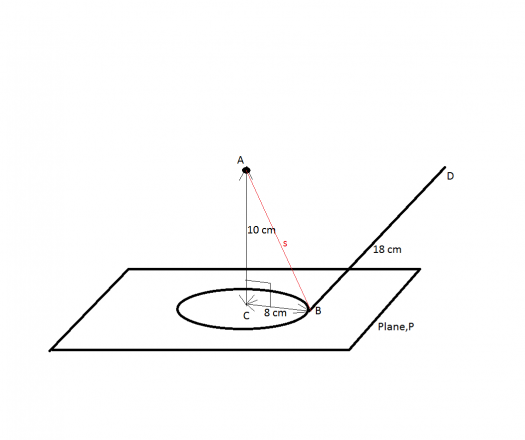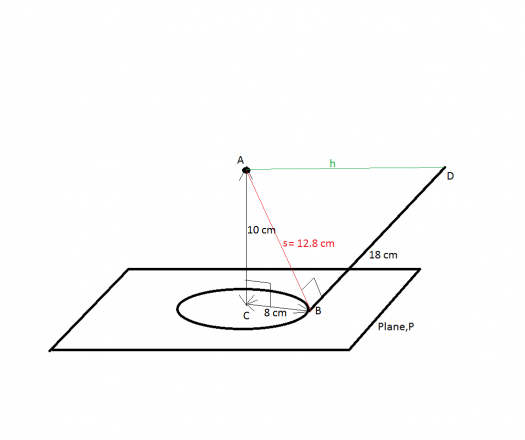2 posts / 0 new
Howard Narvaez Isonfrom a point A 10 cm from a plane P, a perpendicular line AC is drawn passing through a circle with center C and radius of 8 cm in the plane. At any point B on this circle, a tangent BD is drawn 18 cm in length. FInd the distance from A to D.

fitzmerl duronTo answer the problem above, kailangan natin ng drowing....hehehe

The figure that describes the problem looks like this:To get the distance between points $A$ and $D$, we need to get the $s$ first. Using the Pythagorean theorem:$$s^2 = (10 \space cm)^2 + (8 \space cm)^2$$ $$s = 12.8 \space cm$$

Now getting the distance between points $A$ and $D$ (denoted as $h$) using the Pythagorean theorem:$$h^2 = (12.8 \space cm)^2 + (18 \space cm)^2$$ $$h = 22.1 \space cm$$

Therefore, the distance between the points $A$ and $D$ is $\color{green}{22.1 \space cm}$

Alternate solutions are encouraged....heheheh

Subscribe to MATHalino on